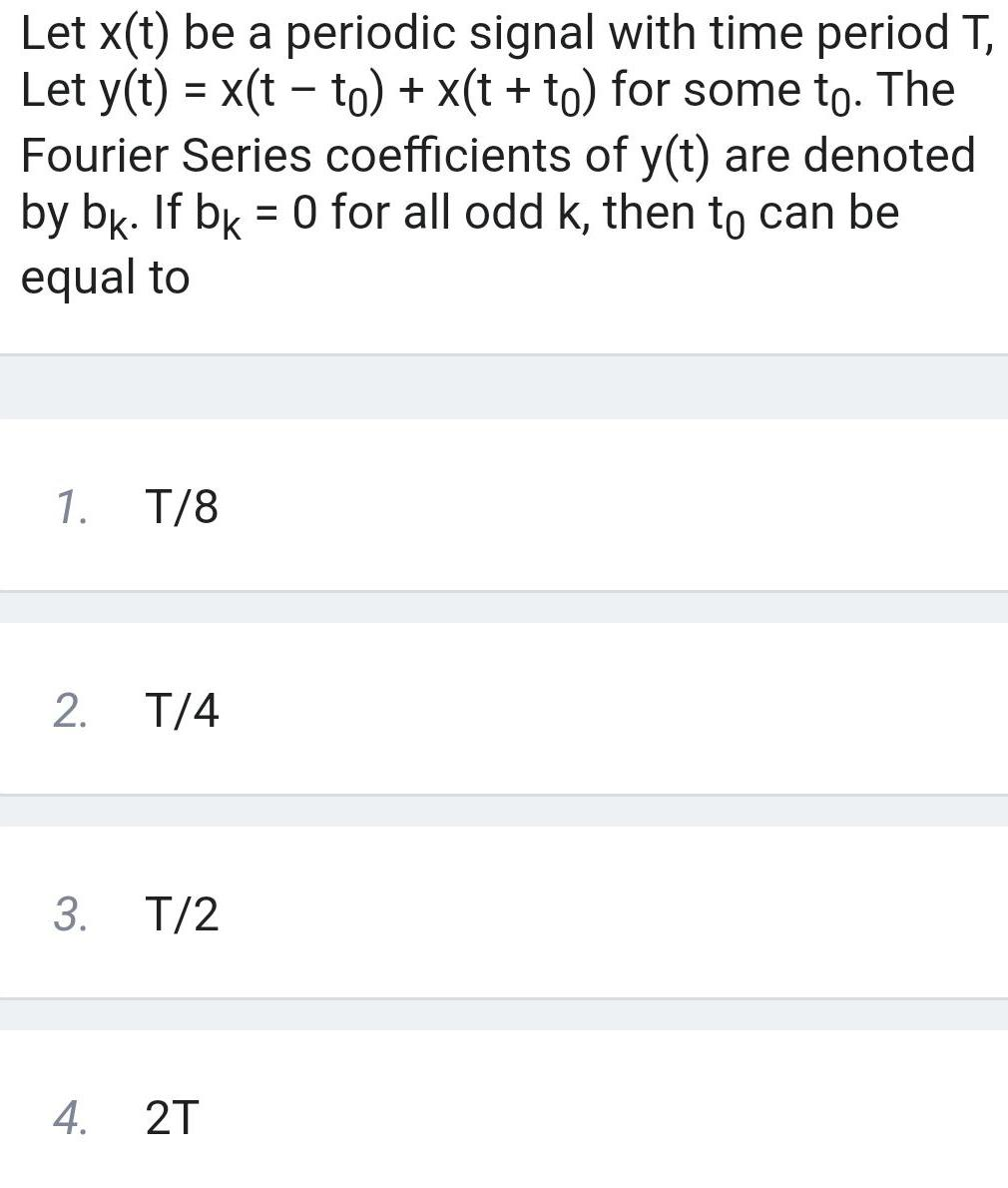Question:

# Let x t be a periodic signal with time period T Let y t x t

Last updated: 6/7/2023Let x t be a periodic signal with time period T Let y t x t to x t to for some to The Fourier Series coefficients of y t are denoted by bk If bk 0 for all odd k then to can be equal to 1 2 3 4 T 8 T 4 T 2 2T